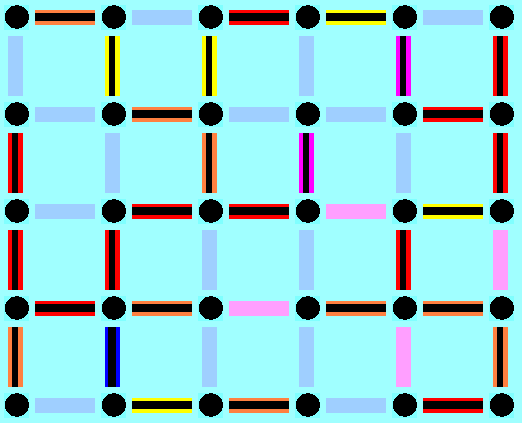CMIS 160
Discrete Mathematics

Erich Prisner
UMUC SG
Spring 2002

# Project #2

This project is due on Thursday, February 28 (firm deadline).

## 1. Lotto

Lotto, "6 aus 49" is a very popular game of chance in Germany. You bet on six numbers out of all numbers between 1 and 49. Then six numbers are chosen randomly, and if they agree with your bet, you are rich. Let us simulate the drawing of the numbers (which in reality is done by choosing six balls mechanically from a large cylinder) using a pseudorandom number generator. Such a generator starts with some "seed" (like actual time), and then gets a sequence of numbers by iteratively applying a function on the seed. Though there is nothing random on these numbers, they may appear quite random.

Here (and on all other pages) we use The Central Randomizer 1.3 (C) 1997 by Paul Houle (houle@msc.cornell.edu). Try to simulate drawings by clicking on the buttons "again", "10 times", or even "100 times". You will see the total of drawings, as well as the number among them obeying certain properties.

Number of weeks
Number of weeks with smallest value between 1 and 9. In percent: %
Number of weeks with two consecutive numbers. In percent: %
Number of weeks where "11" was among the numbers. In percent: %
Number of weeks where all numbers are odd. In percent: %
Number of weeks where all numbers are even. In percent: %

a) Simulate hundreds of drawings and note the percentages for the four events you obtain. Do they make sense to you?

b) How many outcomes of the drawing are possible? Recall that the outcome are six different numbers between 1 and 49 (inclusive), and that the order in which they are drawn is irrelevant---the six numbers are ordered from small to large at the end anyway.

c) How many of the possible drawings have the property that the smallest value is between 1 and 9 (inclusive)? Relate this number to the total number of possible different drawings computed in (b), and compare your result with the number obtained in (a).

d) Do the same for the number of possible drawings containing the number "11".

e) Do the same for the number of possible drawings with all six numbers odd.

f) Do the same for the number of possible drawings containing a pair of consecutive numbers, like in 3, 7, 13, 14, 22, 34.

g) If these theoretical probabilities computed in (c), (d), (e), and (f) are close to the experimental values obtained in (a), then the pseudorandom number generator seems to simulate random numbers closely. However, this is not the case for every pseudorandom number generator. Try the one given in the next page page , and compare the numbers obtained with the theoretical values.

h) Try to find another "good" pseudorandom number generator by replacing the Javascript part on the page mentioned in (g) by your invention,

Go to Project # 3.
Go back to Project # 1.

Projects differ in certain ways from homework. They questions there are more complex, more difficult, have several steps, and sometimes you have to use notions and tools from several chapters to solve them. But in most cases, the theorems and tools learned do not suffice to solve all parts of the project. Rather you have to discover certain facts by yourself. Learning how to make "discoveries" in the field is an important part of the class---in real life you don´t have always a textbook telling you exactly what to do. Of course you are encouraged to use any other material---books, webpages, and so on.